# 0.999... facts for kids

Kids Encyclopedia Facts

0.999... (also written as 0.9, and read as "0 point 9 repeating") is one of the ways the number 1 (one) can be written. Even though it is written like this, no matter how many nines there are before the ellipsis, it is still equal in value to 1.

0.999... is a repeating decimal, which means the digit "9" is repeated forever. It is different from 0.999, which only has three 9s.

0.999... can also be written as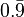$0.9 with bar over the 9$,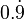$0.9 with dot over the 9$ or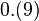$0.(9)$.

It is hard for many people to understand why 0.999... is the same as 1. There are many proofs that show why they are the same number, but many of these proofs are very complex.

## Examples

One simple way of showing that 0.999... and 1 are the same thing is to divide them both by the number 3. When 0.999... is divided by 3, the answer is 0.333..., which is the same as 13 (the fraction one third).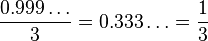${0.999\ldots \over 3} = 0.333\ldots = \frac 13$

When 1 is divided by 3, the answer is 13. Since the answers are the same, that means that 0.999... and 1 are the same. Another way of thinking about it is if 13 = 0.333... and 23 = 0.666..., then 33 = 0.999... therefore, as 33 = 1, 0.999... must also equal 1. There are many other ways of showing this.

Another way of proving that 0.999... = 1 is by accepting the simple fact that if two numbers are different, there must be at least one number between them. For example, a number between 1 and 2 is 1.5, and a number between 0.9 and 1 is 0.95. Since 0.999... has an infinite number of 9s, there cannot be another number after the "last" 9, meaning there is no number between 0.999... and 1. Therefore, they are equal.

One more common proof is such: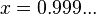$x = 0.999...$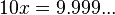$10x = 9.999...$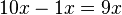$10x - 1x = 9x$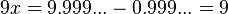$9x = 9.999... - 0.999... = 9$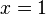$x = 1$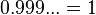$0.999... = 1$

## In popular culture

As the Internet developed, arguments about 0.999... are often on newsgroups and message boards. Even newsgroups and message boards that do not have much to do with math argue about this. In the newsgroup sci.math, arguing about 0.999... is a "popular sport". It is also one of the questions in its FAQ.0.999... Facts for Kids. Kiddle Encyclopedia.